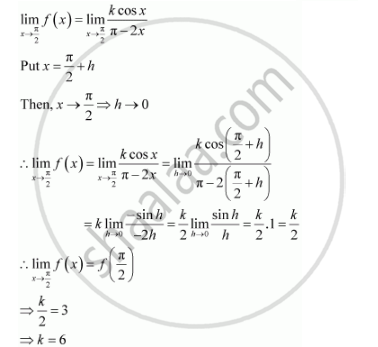Share

# Find the Values Of K So that the Function F Is Continuous at the Indicated Point. F(X) = {((Kcosx)/(Pi-2x), If X != Pi/2),(3, If X = Pi/2) at X Pi/2 - Mathematics

#### Question

Find the values of so that the function f is continuous at the indicated point.

f(x) = {((kcosx)/(pi-2x), "," if x != pi/2),(3, "," if x = pi/2):}  " at x ="  pi/2

#### Solution 1

The given function f is f(x) = {((kcosx)/(pi-2x), "," if x != pi/2),(3, "," if x = pi/2):}  " at x "  pi/2

#### Solution 2

The given function f is f(x) = {((kcosx)/(pi-2x), "," if x != pi/2),(3, "," if x = pi/2):}

The given function f is continuous at ,x= pi/2 if f is defined at x= pi/2and if the value of the f at x= pi/2 equals the limit of f at x= pi/2

It is evident that is defined at x= pi/2 and f(pi/2) = 3Therefore, the required value of k is 6.

Is there an error in this question or solution?